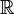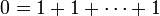# Field (mathematics)

Fields are algebraic structures that generalize on the familiar concepts of real number arithmetic. The set of rational numbers, the set of real numbers and the set of complex numbers are all fields under the usual addition and multiplication operations.

The term field carries other meanings in other areas of mathematics, notably in calculus and mathematical physics. This article deals exclusively with fields as used in abstract algebra.

## Informal description and definition

When dealing with$\mathbb{Q}$, the set of rational numbers, we notice several things:

• The rational numbers form what mathematicians call an abelian group under addition.
• When we exclude the number 0, they form an abelian group under multiplication as well.
• For any triplet a,b,c, we have that a(b + c) = ab + ac - both calculations yield the same answer.

Dealing with other sets, both finite and infinite, we often notice this behavior. Obvious examples are$\mathbb{R}$, the set of real numbers and$\mathbb{C}$, the set of complex numbers.

All these sets, and others where the three conditions listed above are fulfilled, are known as fields.

Less obvious examples are Z2, the set {0,1} under addition and multiplication modulo 2. Other examples are Z3 and Z5, ... , Zp. In general, any set {0,1,...,p-1} under addition and multiplication modulo p, for any prime number p, is a field.

It's important to notice that neither the field elements nor the binary operations necessarily have to be anything closely resembling numbers. It is sufficient that the actual system under study can be conceptualized into a structure that satisfies the formal definition of a field.

## Formal definition of a field

A field consists of a set F, along with a binary operation + on F such that F is a commutative group with an identity element 0; and another binary operation * on F such that F\{0} is a commutative group with identity element 1. Distributivity of * over + holds: that is, for any$a,b,c \in F$, we have that a * (b + c) = a * b + a * c.

The first binary operation is usually called addition and the second one multiplication.

## Characteristic of a field

The characteristic of a field is the smallest natural number k such that$0=1+1+\cdots+1$ (where there are k ones on the right-hand side). If such a natural number does not exist, then the characteristic of a field is taken to be 0. If the characteristic of a field is nonzero, it is a prime number because otherwise, the number$1+1+\cdots+1$, where the number of ones is a proper divisor of the characteristic, would not have an inverse.